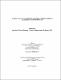## Bi-directional determination of sparse Jacobian matrices : algorithms and lower bounds2015
##### Authors
Saha, Anik
University of Lethbridge. Faculty of Arts and Science
##### Publisher
Lethbridge, Alta. : University of Lethbridge, Dept. of Mathematics and Computer Science
##### Abstract
Efficient estimation of large sparse Jacobian matrices is a requisite in many large-scale scientific and engineering problems. It is known that estimation of non-zeroes of a matrix can be viewed as a graph coloring problem. Due to the presence of dense rows or dense columns, unidirectional partitioning does not always give good results. Bi-Directional partitioning handles the problem of dense rows and dense columns quite well. Lower bound to determine the non-zeroes of a sparse Jacobian matrix can be defined as the least number of groups necessary to determine the matrix. For unidirectional partitioning, a good lower bound is given by the maximum number of non-zeroes in any row. For bi-directional determination, both columns and rows must be considered to obtain a lower bound. In this thesis, we provide an easily computed better lower bound. We have developed a heuristic algorithm and an iterative algorithm to determine non-zeroes of sparse Jacobian matrices using Bi-Directional partitioning. Our heuristic algorithm is inspired from graph coloring problems and recursive largest first partitioning of graphs. Our algorithm provides a better result than the existing algorithms. For the iterative method, we have introduced randomization technique to color the vertices of the graph. A part of our work was presented at "Applied Mathematics, Modelling and Computational Science" (AMMCS-2015).
##### Keywords
sparse matrix , Jacobian matrix , row and column compressions , bi-directional partitioning